Inflation is defined as the process which leads to the price of the price of the goods in the future, as compared to its present value. With inflation, the level of prices increases but money loses its value. Let us try to understand this by taking an example. If the percentage of inflation is 100%, then the cost of an apple will be \$1 the next year, whose price in this current year is \$0.50.

Contracts written in nominal terms fail to represent a real-life situation. Therefore, contracts should be written in real terms by including inflation-indexed terms.

Let us know study the effect of inflations on returns.

5.2.A MEASUREMENT OF THE INFLATION RATE

The Bureau of Labor Statistics came up with a measure of inflation rate, which calculates the number of indexes and fixes the average total price of these bundles on a monthly basis. Consumer Price index is a term given to a hypothetical average index of total household consumption. Other indexes which are commonly used as inflation measures are Producer Price Index and the broader GDP deflator.

When it comes to a contract and the true inflation rate is zero, if the CPI rate is positive, Social Security recipients will receive more from the government. Therefore, government has to pay less when the inflation rate is lower. Factors such as effective price decline of computers and telecommunication products and role of superstores play a crucial role in affecting the rate of inflation.

REVIEW QUESTION

1. Go through the website of Bureau of Labor’s Statistics and compare the CPI and PPI.

5.2. B REAL AND NOMINAL RATES OF INTEREST

A return which has not been adjusted for inflation is called the nominal rate. A return which is calculated as if there is no price inflation to consider is called the real rate of return. Real rate reflects the scenario of inflation, i.e. a dollar will have less purchasing power in the future than it has today. The measure of trade-off between present and future consumption, by considering the change of price is taken care of by real rate of return.

Consider a scenario, where the inflation rate is 100%, and you bought a bond which promises a nominal interest rate of 700%. In order to find the real rate of return, let us assume that the present cost of one apple be \$1. So, you would require \$2 to buy the same apple. With the nominal rate of 700%, the investment of \$1 toady will give \$8 dollars the next year. The next year, with this \$8, you will be able to buy 4 apples and not 8. Therefore the real rate of return is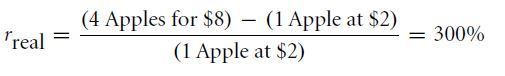Therefore, for every dollar invested today, you get a return of 300% next year, instead of 700% as promised. This is due to the effect of inflation.

The inflation rate can be adjusted by the one-plus formula, which is given by: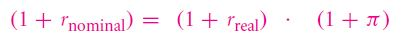The real rate of return is given as: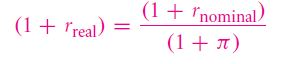If it is said that the future worth of money is more than the present worth of money, it is true only in nominal sense. It should be noted that nominal rates are never negative. Real interest rate can be negative. Let us take an example. If a treasury note is offering 2.58%, and the inflation rate is 3.3%, then the real inflation rate is -0.7% per annum. After 1 year, though it will lead to more cash but with less purchasing power. If there are many goods whose value appreciate with inflation, then there will hardly be any scenario where there will be a negative real rate of return.

REVIEW QUESTION

1. If the nominal rate of return is 20%, inflation is 5%, determine the real interest rate.
1. 2 C NET PRESENT VALUE OF INFLATION

The units of the conversion rate remains the same when it comes to calculate the net present value. The nominal cash flows need to be discounted with nominal discount rates and the real cash flows need to be discounted with real discount rates.

If we refer to the apple example taken in 5.2 B again, we can find out such an arrangement by which we can get 12 apples the next year. All the values remain the same. The cost of an apple this year is \$1, it becomes \$2 the next year, and the nominal interest rate is 700% and the inflation rate is 100%. This can be done by two methods:

1. You need to discount the nominal cash flow, which the 12 apples will yield next year, with the nominal interest rate.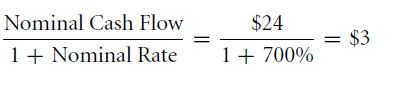1. You need to discount the real cash flow, which the 12 apples will yield the next year, with the real interest rate.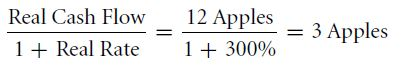The answers is 3 apples in terms of today. If the cost of 1 apple today is \$1, the cost of 12 future apples after a year, today will cost \$3.

Both the methods will give the same NPV. This is because the term of inflation cancels out. The high rate of nominal interest show that the nominal cash flows are less worthy the next year than they are in this year. Nominal interest rates are used more commonly than real interest rates.

REVIEW QUESTION

1. If the real interest rate per annum is 3%, inflation rate is 8% per annum, then find out the present value of an investment which is yielding a nominal value of \$500,000 next year.

Links of Next Financial Accounting Topics:-### Customer Reviews

My Homework Help
Rated 5.0 out of 5 based on 510 customer reviews at